﻿ 基于多重信号分类与可控响应功率的变压器局部放电故障定位方法 Partial Discharge Fault Location Method of Transformer Based on MUSIC-SRP Algorithm

Smart Grid
Vol. 09  No. 06 ( 2019 ), Article ID: 33397 , 19 pages
10.12677/SG.2019.96031

Partial Discharge Fault Location Method of Transformer Based on MUSIC-SRP Algorithm

Liwei Zheng, Wei Wang, Zeqin Lv, Yushuai Zhang, Shijie Wang

Beijing Key Laboratory of High Voltage & EMC, State Key Laboratory of Alternate Electrical Power System with Renewable Energy Sources, North China Electric Power University, Beijing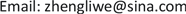Received: Nov. 20th, 2019; accepted: Dec. 4th, 2019; published: Dec. 11th, 2019ABSTRACT

A fault location method for partial discharge of transformer based on multiple signal classification (MUSIC) algorithm and steered power response (SRP) sound source location algorithm is proposed. First of all, MUSIC algorithm is used to measure the turn to turn partial discharge of high and low voltage windings in transformer, considering the error range, the search space of SRP algorithm can be effectively reduced. Then SRP algorithm is used to locate partial discharge in the reduced search space, and the location accuracy of SRP algorithm is significantly improved with the reduction of search space. On this basis, with the application of the fiber-optic ultrasonic sensor array, the localization simulation and experimental results of the partial discharge between turns in the high and low voltage winding of the transformer shows that the localization accuracy of MUSIC-SRP algorithm is greatly improved compared with SRP algorithm, which verifies the effectiveness of the method.

Keywords:Partial Discharge, Fiber-Optic Ultrasonic Sensor, Multiple Signal Classification, Steered Power

Response1. 引言

MUSIC算法作为目前发展成熟、应用广泛且最具代表性的基于高分辨率空间谱估计定位算法，通过提取空间特征值信号子空间和噪声子空间的正交性，声信号阵列测向谱峰空间二维并行搜索声源的位置  。MUSIC算法能有效地对变压器高低压绕组匝间局部放电进行有效测向，但由于单个传感器阵列无法定位。满足交叉定位的最少的传感器数量为两个，但变压器内部结构紧凑，且如果再增加传感器阵列个数，对信号采集设备要求高，后续处理计算设备难以满足实时性，经济成本也过高，难以在实际环境中广泛应用。SRP声源定位算法定位精度高，对分布式传感器阵列实用性，适用于油中局部放电的定位 。但SRP定位算法需要多次迭代，有一定的随机性，搜索空间越大，误差越大，且搜索空间中变压器内部结构复杂，会进一步影响定位精度。

2. 阵列定位算法

2.1. 基于MUSIC算法的超声测向方法

$X\left(t\right)=A\left(\theta ,\phi \right)S\left(t\right)+N\left(t\right)$

X(t)是传感器阵列接收信号， $X\left(t\right)={\left[{x}_{1}\left(t\right),{x}_{2}\left(t\right),\cdots ,{x}_{K}\left(t\right)\right]}^{\text{T}}$

S(t)是信号向量， $S\left(t\right)={\left[{s}_{1}\left(t\right),{s}_{2}\left(t\right),\cdots ,{s}_{K}\left(t\right)\right]}^{\text{T}}$

N(t)是噪声向量， $N\left(t\right)={\left[{n}_{1}\left(t\right),{n}_{2}\left(t\right),\cdots ,{n}_{K}\left(t\right)\right]}^{\text{T}}$

$A\left(\theta ,\phi \right)$ 为信号的方向矩阵， $\theta$ 表示方位角， $\phi$ 表示俯仰角，用 $\left(\theta ,\phi \right)$ 表示信号的DOA，

$A\left(\theta \right)=\left[a\left({\theta }_{1},{\phi }_{1}\right),a\left({\theta }_{2},{\phi }_{2}\right),\cdots ,a\left({\theta }_{K},{\phi }_{K}\right)\right]$

$a\left({\theta }_{i},{\phi }_{i}\right)=\mathrm{exp}\left(j2\pi fr{k}_{i}/v\right)$$i=1,2,\cdots ,K$

${k}_{i}={\left[\mathrm{sin}\left({\phi }_{i}\right)\mathrm{cos}\left({\theta }_{i}\right),\mathrm{sin}\left({\phi }_{i}\right)\mathrm{sin}\left({\theta }_{i}\right),\mathrm{cos}\left({\phi }_{i}\right)\right]}^{\text{T}}$

MUSIC算法的基本思想是对阵列信号的协方差矩阵进行特征分解，根据噪声子空间与信号方向矢量的正交性估计DOA ：

$R=E\left\{X\left(t\right)X{\left(t\right)}^{\text{H}}\right\}=A\left(\theta \right){R}_{S}A{\left(\theta \right)}^{\text{H}}+{\sigma }^{2}I$

$R=U\Sigma {U}^{\text{H}}={U}_{S}{\Sigma }_{S}{U}_{S}^{\text{H}}+{U}_{N}{\Sigma }_{N}{U}_{N}^{\text{H}}$

${U}_{N}^{\text{H}}a\left({\theta }_{k}\right)=0$

${P}_{music}=\frac{1}{{a}^{\text{H}}\left(\theta \right){U}_{N}{U}_{N}^{\text{H}}a\left(\theta \right)}$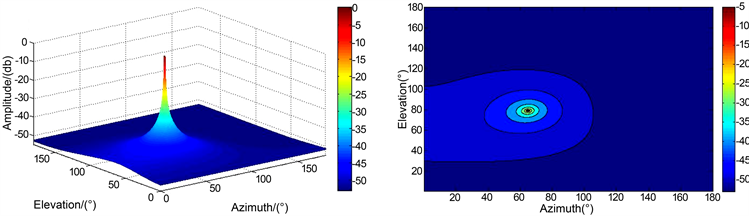Figure 1. MUSIC direction-finding spectrum and contour map

$R=\frac{1}{L}\underset{n=1}{\overset{L}{\sum }}X\left(n\right)$

2.2. 基于SRP算法的超声定位方法

${R}_{mn}\left(\tau \right)=E\left[{x}_{m}\left(t\right){x}_{n}\left(t-\tau \right)\right]$

${R}_{{x}_{m}{x}_{n}}\left(\tau \right)=\frac{1}{2\pi }\underset{-\infty }{\overset{+\infty }{\int }}{\psi }_{mn}\left(w\right){X}_{m}\left(w\right){X}_{n}^{\ast }\left(w\right){\text{e}}^{jw{\tau }_{mn}}\text{d}w$

${\psi }_{mn}\left(w\right)=|\frac{1}{{X}_{m}\left(w\right){X}_{n}^{\ast }\left(w\right)}|$

${\psi }_{mn}\left(w\right)$ 取值为第m个传感器和第n个传感器接收到信号幅度谱的倒数，实际上是一个白化滤波器，能够白化互功率谱，使信号互相关的幅度谱信息得以保留并平滑信号间的互功率谱，提高定位精度。

${\tau }_{mn}\left(s\right)$ 在近场条件下其计算方法为：

${\tau }_{mn}\left(s\right)=\left(‖{r}_{s}-{r}_{m}‖-‖{r}_{s}-{r}_{n}‖\right)/v$

$P\left(z\right)=\underset{m=1}{\overset{M}{\sum }}\underset{n=m+1}{\overset{M}{\sum }}{R}_{{x}_{m}{x}_{n}}\left(\tau \right)$

SRP不仅可以看作是加权的滤波-求和的波束形成，还可以看作是所有传感器对的GCC之和，具有稳健性 。

$\stackrel{^}{s}=\underset{s}{\mathrm{arg}}\mathrm{max}P\left(z\right)$

2.3. MUSIC与SRP相结合的局部放电定位方法

MUSIC测向算法对变压器内高低压绕组匝间局部放电测向时误差较小，适用性较强，可以将SRP的搜索空间合理有效地限定在某一较小范围内。SRP定位算法作为典型的分布式阵列传感器定位算法，可以在搜索空间较小情况下，对空间任意一点进行准确定位。MUSIC-SRP算法结合两种算法的优点，能显著提高局部放电定位精度，核心是利用MUSIC测向算法合理有效地将SRP的搜索空间缩小，使定位精度提升。利用图2分析MUSIC测向算法缩小SRP搜索空间的原理，建立变压器xoz平面坐标系如图3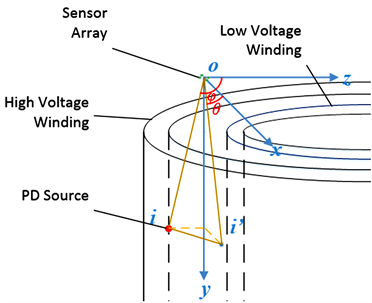Figure 2. Schematic diagram of MUSIC direction finding for turn to turn partial discharge of transformer high voltage winding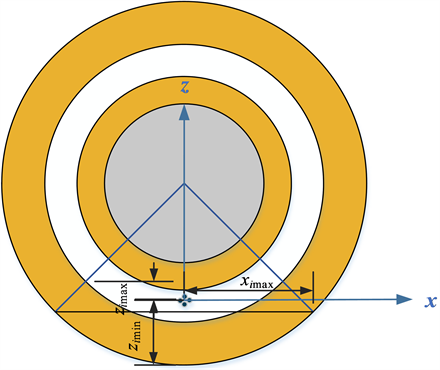Figure 3. Transformer xoz plane coordinate system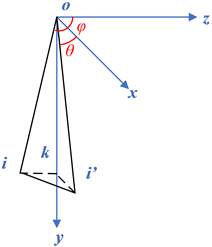Figure 4. Simplified diagram of MUSIC direction-finding

3. 仿真研究

3.1. 仿真模型

$\frac{1}{{c}_{0}^{2}}\frac{{\partial }^{2}p}{\partial {t}^{2}}-{\nabla }^{2}p=0$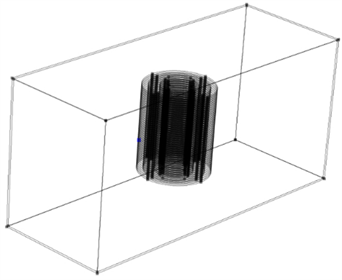Figure 5. Simulation model of transformer oil tank and winding

${f}_{pd}=A{\text{e}}^{-t/\tau }\mathrm{sin}\left(2\pi ft\right)$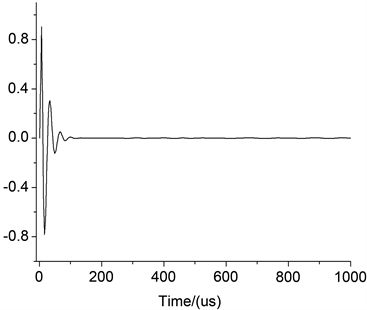Figure 6. Simulation waveform of partial discharge function

3.2. 空间坐标系的建立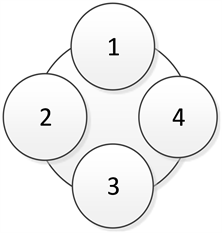Figure 7. Fiber optic FP sensor array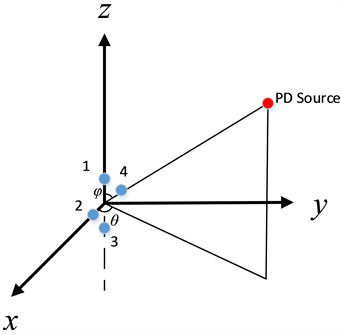Figure 8. Three dimensional coordinate system of space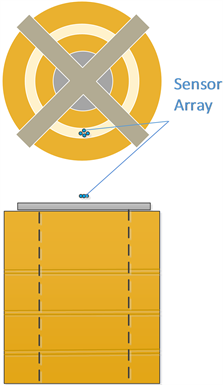Figure 9. Top view and main view of relative position of sensor array and transformer winding

3.3. 局部放电点设置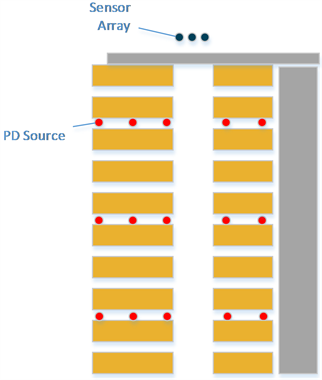Figure 10. Setting of partial discharge points in different depths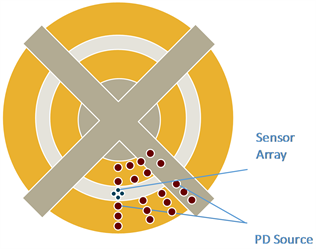Figure 11. Setting of partial discharge points with different deviation angles

3.4. 仿真结果Table 2. Positioning results of MUSIC-SRP algorithm for high voltage upper winding

MUSIC-SRP算法对变压器高压中层绕组匝间局部放电定位结果如表3所示。Table 3. Location results of MUSIC-SRP algorithm for high voltage middle winding

MUSIC-SRP算法对变压器高压下层绕组匝间局部放电定位结果如表4所示。Table 4. Positioning results of MUSIC-SRP algorithm for lower winding of high voltageTable 5. Location results of MUSIC-SRP algorithm for low voltage upper winding

MUSIC-SRP算法对变压器低压中层绕组匝间局部放电定位结果如表6所示。Table 6. Location results of MUSIC-SRP algorithm for low voltage middle winding

MUSIC-SRP算法对变压器低压下层绕组匝间局部放电定位结果如表7所示。Table 7. Location results of MUSIC-SRP algorithm for low voltage lower winding

1) 由于不用阵列形状对某一方向上的测向有不同的误差。因此在放电源对传感器阵列不同角度情况下，产生了不同误差；

2) 由于考虑MUSIC算法测向结果的误差，可能将SRP搜寻范围并没有缩小到最小，产生误差；

3) 由于变压器内部结构复杂，局部放电超声信号传播过程中会发生反射、散射等现象。传感器接收到的超声信号中包含混响信号，这会对局部放电定位产生影响。

4. 实验研究

4.1. 实验平台建立Figure 12. Transformer winding model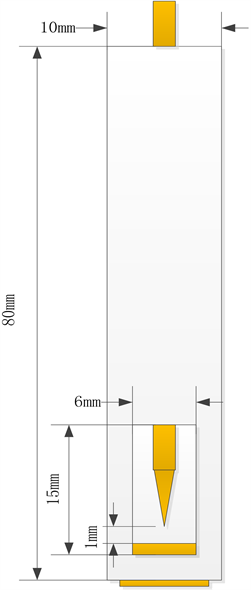Figure 13. Point discharge model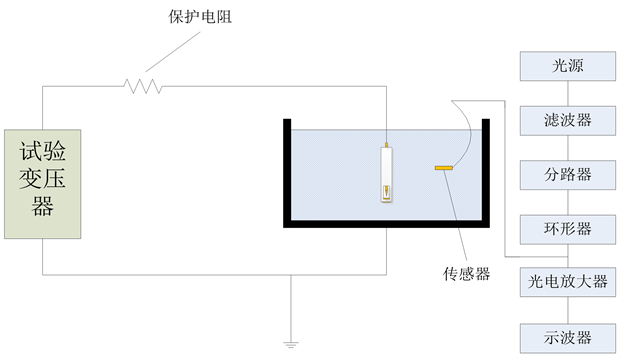Figure 14. Optical fiber sensor test platform of partial discharge

4.2. 结合算法局部放电定位实验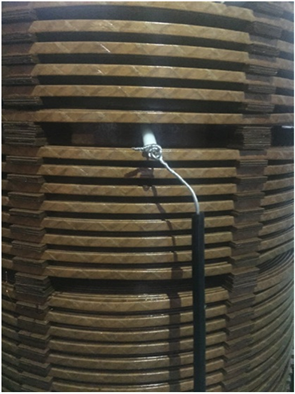Figure 15. Placement of partial discharge power supply in high voltage winding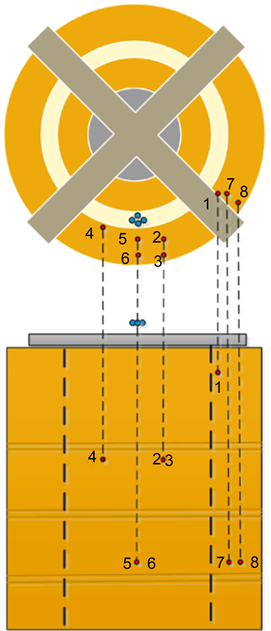Figure 16. Relative position of partial discharge point between turns of high voltage winding

MUSIC-SRP算法对变压器高压绕组匝间局部放电定位实验结果如表8所示。Table 8. Experimental results of MUSIC-SRP algorithm location for high voltage winding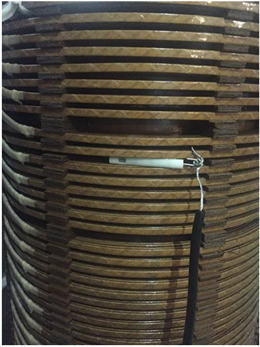Figure 17. Placement of partial discharge power supply in low voltage winding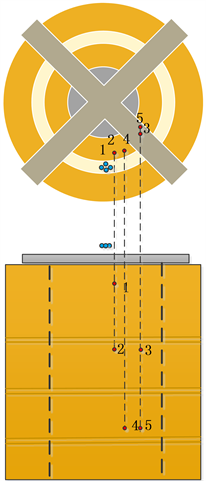Figure 18. Relative position of partial discharge point between turns of low voltage winding

MUSIC-SRP算法对变压器低压绕组匝间局部放电定位结果如表9所示。Table 9. Positioning results of MUSIC-SRP algorithm for low voltage winding

1) 实验环境中存在一些少量的外部噪声，会对对局部放电超声信号波形产生改变，会对定位结果带来些微小误差；

2) 实验中所用的四个光纤FP传感器中心频率需要统一在30 kHz，但实际中四个传感器的中心频率可能会有微小偏差，造成定位误差；

3) 实验过程中的局部放电点坐标都是人为量测设置的，存在量测误差。

5. 结论

Partial Discharge Fault Location Method of Transformer Based on MUSIC-SRP Algorithm[J]. 智能电网, 2019, 09(06): 283-301. https://doi.org/10.12677/SG.2019.96031

1. 1. 王昌长, 李福祺, 高胜友. 电力设备的在线监测与故障诊断[M]. 北京: 清华大学出版社, 2011: 1-3.

2. 2. Mondal, M. and Kumbhar, G.B. (2016) Partial Discharge Localization in a Power Transformer: Methods, Trends, and Future Research. IETE Technical Review, 34, 504-513.
https://doi.org/10.1080/02564602.2016.1209436

3. 3. Jitjing, P., Suwanasri, T. and Suwanasri, C. (2016) The Design of Electrode for Partial Discharge Location Simulation in Oil Insulated Power Transformer and the Application of AE Method. Procedia Computer Science, 86, 289-292.
https://doi.org/10.1016/j.procs.2016.05.074

4. 4. Kunicki, M., Cichoń, A. and Borucki, S. (2016) Study on Descriptors of Acoustic Emission Signals Generated by Partial Discharges under Laboratory Conditions and in On-Site Electrical Power Transformer. Archives of Acoustics, 41, 265-276.
https://doi.org/10.1515/aoa-2016-0026

5. 5. Al-Masri, W.M.F., Abdel-Hafez, M.F. and El-Hag, A.H. (2015) A Novel Bias Detection Technique for Partial Discharge Localization in Oil Insulation System. IEEE Transactions on Instrumentation & Measurement, 65, 448-457.
https://doi.org/10.1109/TIM.2015.2482259

6. 6. 刘化龙. 变压器局放超声检测和定位技术现状及发展[J]. 重庆理工大学学报: 自然科学版, 2014(7): 71-79.

7. 7. 罗勇芬, 李彦明, 刘丽春. 变压器局部放电的超声波和射频联合检测技术的现状和发展[J]. 变压器, 2003, 40(12): 28-31.

8. 8. 罗勇芬, 李彦明. 用于油中局部放电定位的超声相控接收阵传感器的研究[J]. 西安交通大学学报, 2005, 39(4): 402-406.

9. 9. Luo, Y.F., Ji, S.C. and Li, Y.M. (2006) Phased-Ultrasonic Receiving-Planar Array Transducer for Partial Discharge Location in Transformer. IEEE Transactions on Ultrasonics Ferroelectrics & Frequency Control, 53, 614-622.
https://doi.org/10.1109/TUFFC.2006.1610570

10. 10. Xin, X., Luo, Y., Tang, X., et al. (2014) Relocatable Ultrasonic Array and UHF Combined Sensor Applied to PD Location in Oil. IEEE Sensors Journal, 14, 357-361.
https://doi.org/10.1109/JSEN.2013.2283156

11. 11. 辛晓虎, 罗勇芬, 杜非, 等. 用于油中局部放电定位的超声波接收阵列的定向算法比较[J]. 中国电机工程学报, 2015, 35(20): 5351-5359.

12. 12. 谢庆, 律方成, 郑书生, 等. 利用超声相控阵的油中局部放电定位实验分析[J]. 高电压技术, 2010, 36(11): 2711-2716.

13. 13. Judd, M.D. and Farish, O. (2000) Power Transformer Monitoring Using UHF Sensors: Installation and Testing. In: Proceedings of the 2000 IEEE International Symposium on Electrical Insulation, IEEE, Anaheim, 373-376.

14. 14. Judd, M.D. and Cleary, G.P. (2002) Power Transformer Monitoring Using UHF Sensors: Site Trials Conference. Proceedings of the 2002 IEEE International Symposium on Electrical Insulation, IEEE, Boston, 145-149.

15. 15. 唐志国, 李成榕, 黄兴泉, 等. 基于辐射电磁波检测的电力变压器局部放电定位研究[J]. 中国电机工程学报, 2006, 26(3): 17-21.

16. 16. 罗日成, 李卫国, 李成榕. 基于阵列信号处理的变压器内局部放电源多目标定位方法[J]. 电网技术, 2006, 30(1): 65-69.

17. 17. Dibiase, J.H. (2000) A High-Accuracy, Low-Latency Technique for Talker Localization in Reverberant Environments Using Microphone Arrays. European Journal of Biochemistry, 216, 281-291.

18. 18. Cai, W., Wang, S. and Wu, Z.Y. (2010) Accelerated Steered Response Power Method for Sound Source Localization Using Orthogonal Linear Array. Applied Acoustics, 71, 134-139.
https://doi.org/10.1016/j.apacoust.2009.07.015